# C++ Template: The Complete Guide

1
92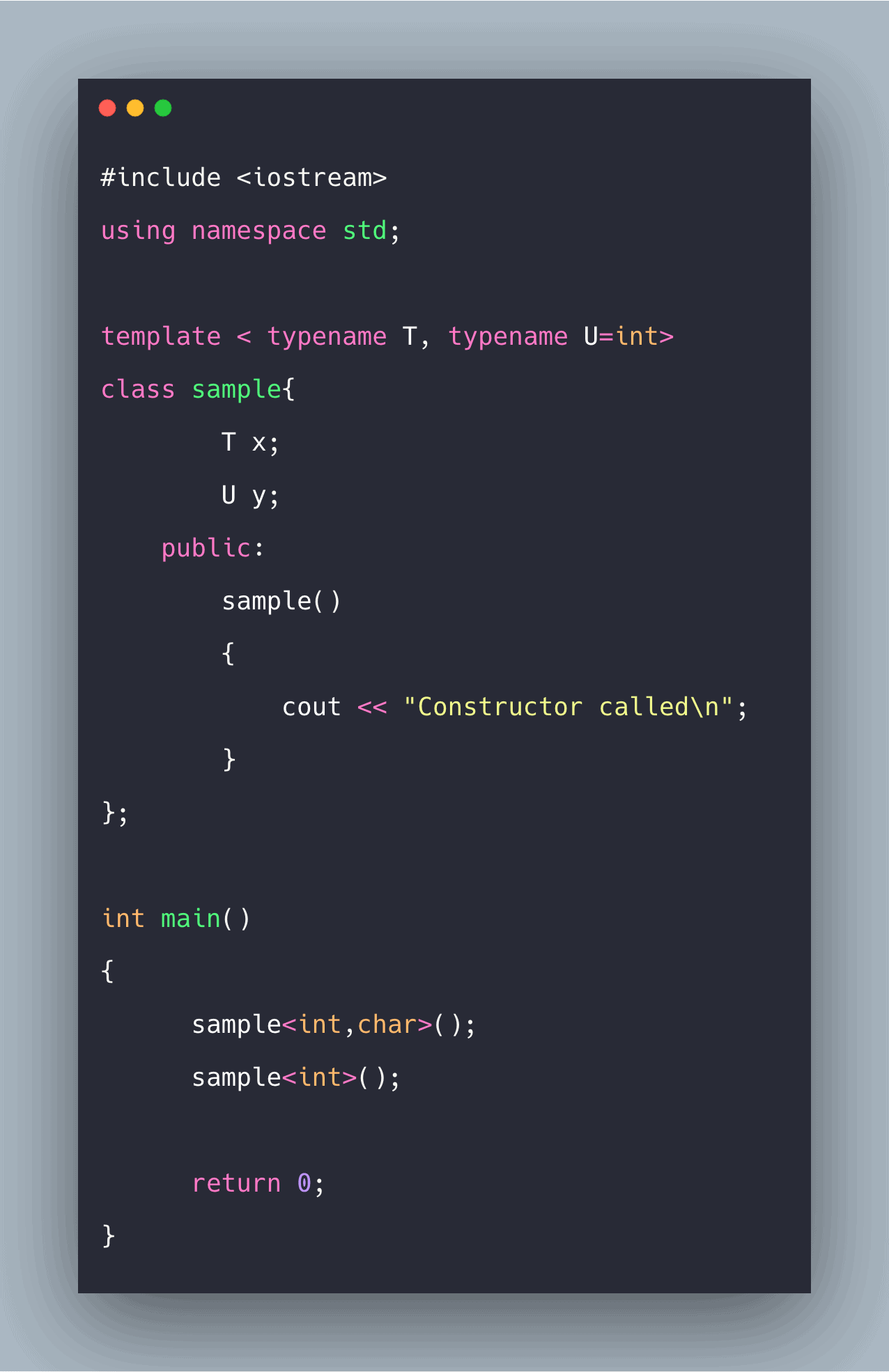C++ templates are a simple yet powerful tool because the idea is to pass data type as the parameter so that we don’t need to write the same code for different data types.

Templates make your classes more abstract by letting you define the class’s behavior without knowing how the class operations will handle data type.

## C++ Template

Templates in C++ allow you to write generic programs. Templates can be used in conjunction with abstract data types to enable them to handle any data.

Using templates, you can create a single function or a class to work with different data types.

For example, you could make the templated stack class that can handle a stack of any data type rather than creating the stack class for every different datatype for which you want the stack to function.

A class template provides the specification for generating classes based on parameters.

Class templates are generally used to implement the containers. A class template is instantiated by passing the given types as template arguments.

## Why use templates in C++?

Templates are used for generic programming. Generic programming means the same codes can be used for different input data types.

This will reduce the number of lines of code because the same function or class can be used for various data types.

Two keywords, namely template and typename or class, are used to write templates in C++.

## How do C++ templates work?

At compile time compiler generates the same template function for different data types used in the main function.

Source code will contain only the template function/class but compiled code will provide copies of the same function/class based on different data types.

## Sample template function in C++

We must find the sum of all the numbers in an array. Inputs to the array can be integer or float. So here template function will be made, which can take both integer array and floating array as input and outputs the sum of the numbers present.

Instead of making two separate functions, one for integer and one for float, we will settle with only one that is a template function.

To write the template function for summation, it is written typically, and the changes are present in the return type and input parameters data type if it has to be generic.

We can define the generic data type by saying “T” and use the keyword class or typename just before writing the main function. So, the typename <class T > is written just above the function.

Now, “T” can be used in place of data types to be defined. So, if you want int a to be generic, write T a, and we are done. See the following example of sampletemplate.cpp file.

```#include <iostream>
using namespace std;

template < class T >
T sum(T a[],int sz)
{
int i;
T ans=0;
for(i=0;i<sz;i++)
ans += a[i];
return ans;
}

int main()
{
// Sample use of template function
int a = {1,2,3,4};
float b = {1.2,0.8,1.2,10.1,10.2};

cout << "Integer array sum : " << sum<int>(a,4) << "\n";
cout << "Float array sum : " << sum<float>(b,5) << "\n";

return 0;
}```

See the following output.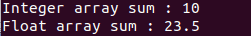Template functions for sort(), max(),min() can also be implemented in similar way.

## Sample template class

Similar to the template function, we can also implement a template class. Template classes can be made for data structures like an array, linked list, queue, stack, tree, etc.

### Template using one datatype:

Below is the sample template class of stack with its basic functions push() and pop(). It can be used for any data type.

Before defining the class, typename < typename T> is written to make it a template class.

Now ‘T’ can be used inside the class as the generic data type. Like in the stack class, we can use ‘T’ to define a generic array.

It is merely like suppose you write a simple stack class for integer values and then replace data types of variables associated with elements of a stack with “T”.

```#include <iostream>
using namespace std;

template < typename T >
class stack_
{
T * arr;
int sz; // size
int num_of_elements;
public:
stack_(int s)
{
arr = new T[s];
sz=s;
num_of_elements = 0; // zero elements at start
}

void push(T x)
{
if(num_of_elements == sz)
{
cout << "Stack Full : Can't be inserted\n";
return;
}

arr[num_of_elements] = x;
num_of_elements++;
}
T pop()
{
if(num_of_elements == 0)
{
cout << "Stack Empty : Can't be popped\n";
return -1;
}
T value = arr[num_of_elements-1];
num_of_elements--;
return value;
}
};
int main()
{
// Sample use of stack template class
stack_<int> s(5);
s.push(1);
s.push(2);
s.push(3);
s.push(4);
s.push(5);
int v = s.pop();
cout << v << "\n";

return 0;
}```

See the following output.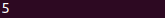### Template using two different datatypes

Also, we can have more than one data type in template functions or classes.

Suppose we have to make a function dealing with two different data types. A generic template function can be used to make these two different data types.

So, instead of template<typename T>, we would use template<typename T, typename U> to tell a compiler that we will use two generic data types that may differ.

Once this line is written, we can start writing the function using datatype “T” and datatype “U” where ever required.

```#include <iostream>
using namespace std;

template < typename T, typename U>
void sum(T a[],int n,U b[],int m)
{
// two different types of array can be passed
T sum_type1 = 0;
int i;
for(i=0;i<n;i++)
sum_type1 += a[i];

cout << "Sum of Array of Type 1 : " << sum_type1 << "\n";

U sum_type2 =0;
for(i=0;i<m;i++)
sum_type2 += b[i];
cout << "Sum of Array of Type 2 : " << sum_type2 << "\n";

}

int main()
{
// Sample use of template function with two data types

int a = {1,2,3};
float b = {3.6,5.1,4.5};

sum<int,float>(a,3,b,3);

return 0;
}```

See the following example.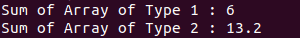### Template using default datatype

Since we are using more than datatypes, we can set some of them as the default, but they must be present at last.

So if we make an instance of this template class, we can instantiate with fewer data types if the default datatype had been at the time of defining the template class. In template < typename T,typename U=int> ‘U’ has been set to default int.

So if we make an instance with just one data type, there will be no problem, and the constructor will be called in that case also because we have set the other generic data type that is ‘U’ to default, and it will automatically assume it to be of int type.

See the following code.

```#include <iostream>
using namespace std;

template < typename T, typename U=int>
class sample{
T x;
U y;
public:
sample()
{
cout << "Constructor called\n";
}
};

int main()
{
// Sample use of template function with two data types
sample<int,char>();
sample<int>();

return 0;
}```

See the following output.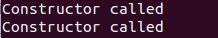That’s it.

#### 1 Comment

1.oreillys store hours

Outstanding post, you have pointed out some good points,
I likewise conceive this is a very good website.

This site uses Akismet to reduce spam. Learn how your comment data is processed.